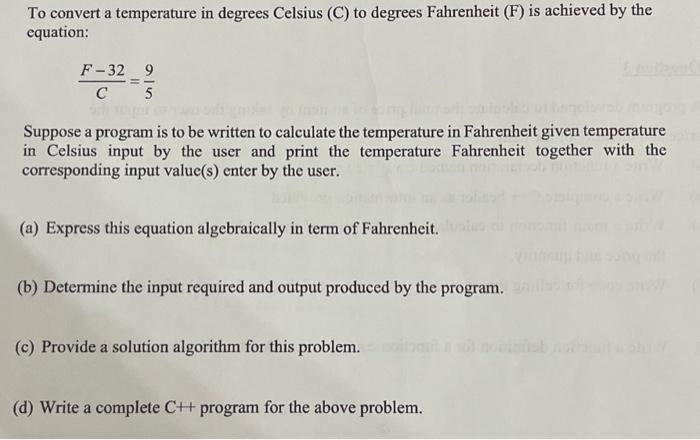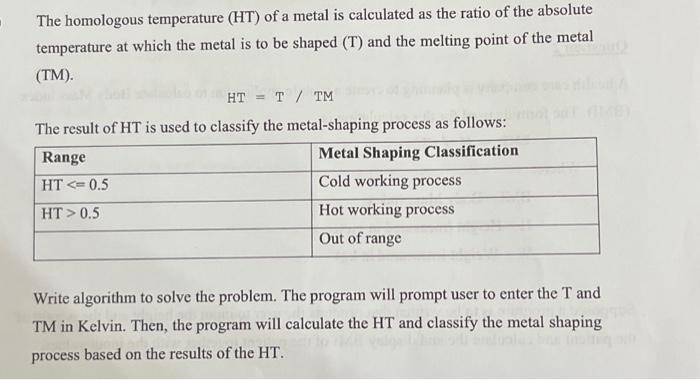# (Solved): Please help me with this using C++ Programbing method Will give , cheers! To convert a temperatu ...

Will give ????, cheers!To convert a temperature in degrees Celsius (C) to degrees Fahrenheit is achieved by the equation: Suppose a program is to be written to calculate the temperature in Fahrenheit given temperature in Celsius input by the user and print the temperature Fahrenheit together with the corresponding input value(s) enter by the user. (a) Express this equation algebraically in term of Fahrenheit. (b) Determine the input required and output produced by the program. (c) Provide a solution algorithm for this problem. (d) Write a complete program for the above problem. The homologous temperature of a metal is calculated as the ratio of the absolute temperature at which the metal is to be shaped and the melting point of the metal . The result of HT is used to classify the metal-shaping process as follows: Write algorithm to solve the problem. The program will prompt user to enter the and TM in Kelvin. Then, the program will calculate the HT and classify the metal shaping process based on the results of the HT.

We have an Answer from Expert

(a) Expressing the equation algebraically in terms of Fahrenheit:

Multiplying both sides by C:

1. The equation   represents the relationship between Fahrenheit (F) and Celsius (C) temperatures.
2. To isolate F, we multiply both sides of the equation by C to get rid of the denominator on the left side.
3. This gives us
4. Next, we add 32 to both sides of the equation to move the -32 term to the other side.
5. This results in
6. Now, the equation is expressed algebraically in terms of Fahrenheit (F) alone.
7. This new equation can be used to calculate the temperature in Fahrenheit when the temperature in Celsius is known.
8. The    part represents the conversion factor from Celsius to Fahrenheit.
9. Adding 32 at the end accounts for the offset between the two temperature scales.
10. Thus,   gives the Fahrenheit temperature corresponding to a given Celsius temperature.

We have an Answer from Expert